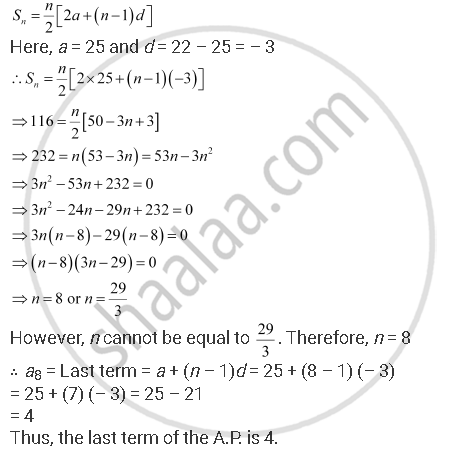CBSE (Arts) Class 11CBSE
Share

# If the sum of a certain number of terms of the A.P. 25, 22, 19, … is 116. Find the last term - CBSE (Arts) Class 11 - Mathematics

ConceptArithmetic Progression (A.P.)

#### Question

If the sum of a certain number of terms of the A.P. 25, 22, 19, … is 116. Find the last term

#### Solution 1

Let the sum of n terms of the given A.P. be 116.#### Solution 2

In the given problem, we have the sum of the certain number of terms of an A.P. and we need to find the last term for that A.P.

So here, let us first find the number of terms whose sum is 116. For that, we will use the formula,

S_n = n/2[2a+ (n-1)d]

Where; a = first term for the given A.P.

d = common difference of the given A.P.

= number of terms

So for the given A.P (25, 22, 19, .....)

The first term (a) = 25

The sum of n terms S_n = 116

Common difference of the A.P (d) = a_2 - a_1

= 22 - 25

= -3

So, on substituting the values in the formula for the sum of n terms of A.P. we get

116 = n/2 [2(25) + (n - 1)(-3)]

116 = (n/2)[50 + (-3n + 3)]

116 = (n/2) [53 - 3n]

(116)(2) = 53n - 3n^2

So, we get the following quadratic equation,

3n^2 - 53n + 232 = 0

On solving by splitting the middle term,we get

3n^2 - 24n - 29n + 232 = 0

3n(n - 8) - 29(n - 8) = 0

(3n - 29)(n - 8) = 0

Further

3n - 29 = 0

n = 29/3

Also

n - 8 = 0

Now since n cannot be a fraction, so the number of terms is a8

a_8 = a_1 + 7d

= 25 + 7(-3)

= 25 - 21

= 4

Therefore the last term of the given A.P such that the sum of terms is 116 is 4

Is there an error in this question or solution?

#### APPEARS IN

NCERT Solution for Mathematics Textbook for Class 11 (2018 to Current)
Chapter 9: Sequences and Series
Q: 6 | Page no. 185

#### Video TutorialsVIEW ALL 

Solution If the sum of a certain number of terms of the A.P. 25, 22, 19, … is 116. Find the last term Concept: Arithmetic Progression (A.P.).
S